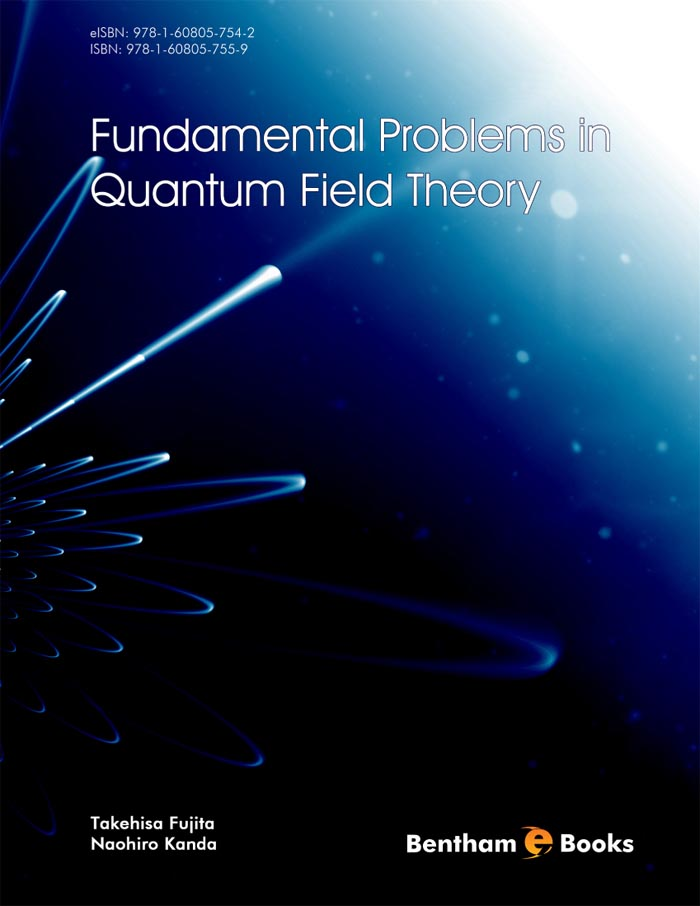### S-Matrix Theory

#### Abstract

In this chapter, we discuss the S-matrix theory in quantum field theory. Here, we first treat the non-relativistic scattering theory and its relation to the Tmatrix. In particular, we discuss the scattering problem in terms of the Lippmann- Schwinger equation. Then we discuss the S-matrix theory in quantum field theory. This is based on the perturbation theory and we present the example of the S-matrix evaluation. In particular, we discuss some basic problems in the Feynman propagator of photon and show a possible physical difference between Feynman and correct propagators of photon.

Total Pages: 20-34 (15)